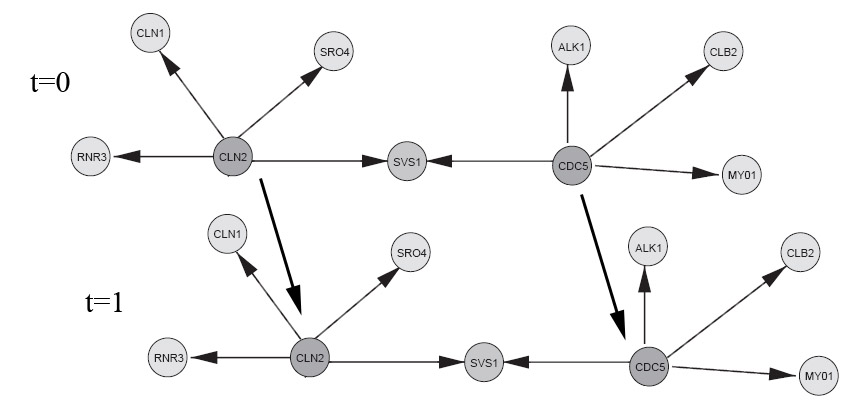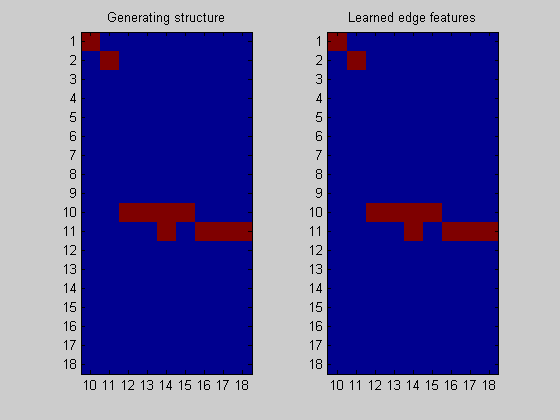## Contents

```% a simple demo of how to do structure learning with data from a DBN
```

## Demo configuration

```rand('seed',0); % Deterministic randomness
randn('seed',0);

HMM = 1;
HUSMEIER = 2;

dbnType = HUSMEIER;
```

## Create a DBN

```if dbnType == HMM

elseif dbnType == HUSMEIER;

% Reproduced from a subset of the network from figure 3, Husmeier.
% "Sensitivity and specificity of inferring genetic
% regulatory interactions from microarray experiments with dynamic
% Bayesian networks". Bioinformatics, Vol. 19 no. 17 2003, pages
% 2271–2282.
% See "husmeier_network.jpg" image in Demos directory to see network
% topology (compare to Husmeier, figure 3).

% show the ground truth
figure('Position',[100 100 size(groundTruthImage,2) size(groundTruthImage,1)]);
image(groundTruthImage); axis('image'); axis('off'); set(gca,'Position',[0 0 1 1])
title('Husmeier ground truth');

% With this choice of inter-slice connections, we can expect to learn
% the full casual bayes-net with observational-only data (assuming the
% sampled parameters induce a faithful distribution).

CLN2 = 1;
CDC5 = 2;
RNR3 = 3;
SRO4 = 6;
CLN1 = 4;
SVS1 = 5;
ALK1 = 7;
CLB2 = 8;
MY01 = 9;

% There appears to be a bug in BNT mk_bnet automatically sorts nodes in topological order, while mk_dbn
% does not (on the intra adjacency matrix)

% From Husmeier's paper
intra = zeros(9);
intra(CLN2,CLN1)=1;
intra(CLN2,SRO4)=1;
intra(CLN2,SVS1)=1;
intra(CLN2,RNR3)=1;
intra(CDC5,SVS1)=1;
intra(CDC5,ALK1)=1;
intra(CDC5,CLB2)=1;
intra(CDC5,MY01)=1;

% Add some arbitrarily chosen inter-slice edges
inter = zeros(9);
inter(CLN2,CLN2)=1;
inter(CDC5,CDC5)=1;

dnodes = 1:9;
bnet = mk_dbn(intra, inter, 2*ones(1,9), 'discrete', dnodes);

% sample parameters for the network
bnet = myMkBnet( length(bnet.CPD), bnet.node_sizes(:), 'bnet', bnet, 'method', 'meek');

maxFanIn = 4;
nData = 5000;
end
```## Sample data from the DBN

```data = cell2mat(sample_dbn(bnet, 'length', nData )); % sample some data from the DBN
```

## Convert the data representation to one suitable for direct input into the BNSL routines

Transform the sampled data into a format suitable for BNSL (from time-series to time-slices) Returns a struct holding the new (doubled) # of nodes, a layering that encodes the flow of time, and the transformed data.

```dataDbn = transformDbnData(data, 'maxFanIn', maxFanIn); % *** Important function
```

## Learn the DBN back with the DP algorithm

Since all the parameters are randomly sampled, they could lead to a non-faithful distrubtion (ie. one that encodes conditionally independences not encoded by the DBN).

```% These are the same functions used in simpleDemo.m (they are used throughout the BNSL package)
ep = computeAllEdgeProb( aflp, aflml);

% There is no point in doing structure learning on the first time step, since
% we cannot learn anything from a single data point (in terms of posterior features, such as edges)
% aflp = mkAllFamilyLogPrior( dataDbn.nNodes );
% aflml = mkAllFamilyLogMargLik( data(:,1), 'nodeArity', 2*ones(1,2));
% ep0 = computeAllEdgeProb( aflp, aflml);
```
```Node 1/18
Node 2/18
Node 3/18
Node 4/18
Node 5/18
Node 6/18
Node 7/18
Node 8/18
Node 9/18
Node 10/18
Node 11/18
Node 12/18
Node 13/18
Node 14/18
Node 15/18
Node 16/18
Node 17/18
Node 18/18
```

## Plot the result

```nNodes = length(bnet.intra);
figure;
subplot(1,2,1); imagesc(bnet.dag(:,nNodes+1:end)); title('Generating structure'); axis('equal'); axis('tight');